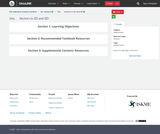# Search Results (2)

View
Selected filters:
• PhysicsConditions of Use:
Remix and Share
Rating

Sinusoidal function, harmonic motion, periodic functions, applications.TMM 002 PRECALCULUS (Revised March 21, 2017)1. Functions: 1a. Analyze functions. Routine analysis includes discussion of domain, range, zeros, general function behavior (increasing, decreasing, extrema, etc.), as well as periodic characteristics such as period, frequency, phase shift, and amplitude. In addition to performing rote processes, the student can articulate reasons for choosing a particular process, recognize function families and anticipate behavior, and explain the implementation of a process (e.g., why certain real numbers are excluded from the domain of a given function).*

Subject:
Mathematics
Calculus
Trigonometry
Physics
Material Type:
Module
Provider:
Ohio Open Ed CollaborativeConditions of Use:
Remix and Share
Rating

Vectors - magnitude, direction, component form, trigonometric form, unit vector, algebra of vectors, applications,TMM 002 PRECALCULUS (Revised March 21, 2017)AdditionalOptional Learning Outcomes:2. Geometry: The successful Precalculus student can:2e. Interpret the result of vector computations geometrically and within the confines of a particular applied context (e.g., forces).Sample Tasks:The student can define vectors, their arithmetic, their representation, and interpretations.The student can decompose vectors into normal and parallel components.The student can interpret the result of a vector computation as a change in location in the plane or as the net force acting on an object.

Subject:
Higher Education
Mathematics
Calculus
Geometry
Physics
Material Type:
Module
Provider:
Ohio Open Ed Collaborative